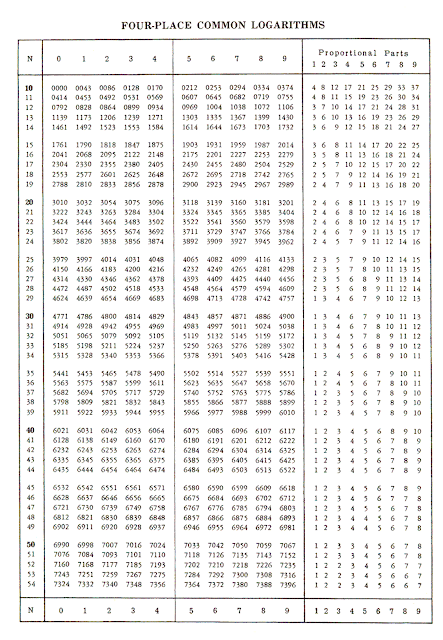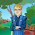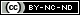## Thursday, December 10, 2020

### Slides and Logs

I believe that learning the “old ways” of doing things has a place in prepping. Knowing how to do things without the aid of modern gadgets will give you more options for the serious SHTF scenarios, such as having to do without electricity anywhere nearby for months or years. Building or rebuilding anything large takes math, that's just a fact of life, and so the more math you understand the better. I'm not saying you have to memorize everything; just knowing how it works and where to find it is a big step towards using it.

50-odd years ago, computers were in their infancy; an average smartphone of today has more memory and computing power than the largest supercomputer of the 1960s. The engineers, machinists, astronomers, and planners of the time all used analog equipment rather than digital. One of the main analog computing devices of the era was the slide rule, a pocket calculator that didn't require batteries and could give quick, accurate results as long as you know how to use it properly. Sadly, the last maker of slide rules went out of business many years ago, but so many millions of them had been made in the hundreds of years prior that you can still find them if you look around.

Slide rules work by using logarithmic scales on two parts, one fixed and the other movable, to perform rapid multiplication and division. Let me explain logarithms first, and then I'll get back to slide rules.

Logarithms
Logarithms are a mathematical function. The easiest way to understand common logarithms (I'll use the shorthand “log”) is to ask, “what exponent of 10 will equal this number?”

If you're not sure what an exponent is, it's the little number placed above and to the right of a number to express a large number in a shorter way (I'm not sure if all of the possible browsers out there can interpret superscript so I'll use an alternate method of denoting exponents: instead of 2² I'll use 2^2.) 4 is 2^2, 32 is 2^5, and 4096 is 2^12.

The nice thing about exponents is that multiplication of numbers is the same as the addition of their exponents as long as you are using the same base number. So to multiply 4096 by 32, you could just add their exponents (5+12=17) and look up the results on a table, which gives you 131,072. Division is the opposite of multiplication, so you can perform that function by subtracting exponents.

Using a log table takes some practice, but once you get the hang of it it's a lot easier than filling pages of paper with long multiplication or division of large numbers. I had to dig out one of my older reference books to find a good log table; most of them are carried out to 4 or 5 decimal places, but I've seen tables much larger.

Here's a sample of a log table.https://www.abelard.org/sums/four_figure_log_tables.php

Using a log table starts with simplifying your large number. To use the example above, 4096 is expressed as 4.096 * 10^3. Simplifying your large numbers to “scientific notation,” aka a number less than 10 times some exponential power of 10 is essential. Log tables only cover the numbers from 0 to 10 because once you go past 10, they repeat.

Since adding logs is the same as multiplying the numbers, you end up with:

log 4.096 + log 10^3

since we're working with the base of 10, the log of 10^3 is 3, and looking at a table tells me that the log of 4.096 is 0.6124. That makes the log 4096=3.6124

Doing the same to 32 gives me: log 32=1.5051

Adding 3.6124 and 1.5051 equals 5.1175, which is 10^5 (100,000) times whatever has a log of 0.1175. Looking that up on the same table tells me that 1.3107 has a log of 0.1175, so our answer is 1.3107 times 100,000 or 131,070. Not exactly the same as 131,072 but it's close enough for most work. Using log tables carried out to more digits will get you closer to the exact number, but this is far less than 1% error (0.000015 to be exact) and was good enough for engineers for a couple hundred years.

Slide Rules
If you're still with me it gets easier. People who worked with math a lot didn't want to have to go back and forth to a table every time they multiplied or divided a large number, so they engraved a logarithmic scale on two pieces of wood, and by sliding the two scales against each other they could mechanize the addition of the logs and read out an approximate answer. This is basically a slide rule. Using the scales engraved on the bars eliminates the need for tables, and as long as you kept your decimal points straight, the answers were within acceptable ranges.Here's a picture of the logarithmic scales and how they're used. In this example they're multiplying 2*3, so they lined up the “2” on the bottom scale with the beginning of the top scale and by “adding” the “3” from the top scale they see that the answer is “6” on the bottom scale. Once you have the bottom scale set, you can get answers to several equations just by looking top scale and following it back down to the bottom. This is why a lot of slide rules had a clear slider with a thin line, it lets you see the answer on the bottom scale a little quicker and also allows for easy follow up equations. Look and you'll see that 2 * 1.5 lines up with 3, 2 * 2.5 lines up with 5, and so on.

Yes, I have slide rules. Most of them have other functions and scales that are more complex than I care to cover here, but the trigonometric functions are handy if you have them. For a cordless, battery-free calculator, they will work long after your cell phone has died and will still give good enough results to put a man on the moon. Follow the link under the picture above for a good article on slide rules and their history.

1.We got to the moon using sliderules. I wish I still had my old Duplex log-log decitrig.

2.I,too, have slide rules and still use them. A very complete bit of information about them can be found here: https://sliderulemuseum.com/SR_Course.htm . If you can find one I recommend the Documentia Geigy "Scientific Tables" book. They used to be given out free to Pharmacy and Med Students when they graduated, which is how I got mine. A quick google shows it is available. Another book is the Merck Index (this is not the Merck Manual, which is about disease and good to have also) which is a compendia of chemicals, compounds, drugs: their properties, how they are produced, and what they are used for.

## The Fine Print